# Performing Table Joins (PyQGIS)¶

This tutorial shows how to use Python scripting in QGIS (PyQGIS) to perform a table join and apply a graduated style to the resulting layer. This tutorial replicates the steps of the Performing Table Joins tutorial using only python scripting.

## Aufgabenübersicht¶

Please refer to Performing Table Joins tutorial for the overview.

### Andere Fähigkeiten, die Sie erlernen¶

• Loading zipped layers in QGIS via Python.
• Using QgsGraduatedSymbolRendererV2 to apply a graduated style to a vector layer.

## Daten besorgen¶

tl_2013_06_tract.zip

ca_tracts_pop.csv

ca_tracts_pop.csvt

Data Source [TIGER] [USCENSUS]

## Arbeitsablauf¶

You can type the following commands in the Python Console or the built-in Editor in QGIS.

1. Load the shapefile. The Census Tracts file is a zip file containing the shapefile. While we can unzip it and load the shapefile, The OGR provider has the ability to load the zip file directly via a Virtual Filesystem. Adding `/vsizip/` in the path, we can access the shapefile contained in the zip archive.

Bemerkung

The zip_uri would begin with `/vsizip//` on Linux and Mac systems. (Note the extra /)

```zip_uri = '/vsizip/C:/Users/Ujaval/Downloads/tl_2013_06_tract.zip'
shp =  QgsVectorLayer(zip_uri, 'tl_2013_06_tract', 'ogr')
```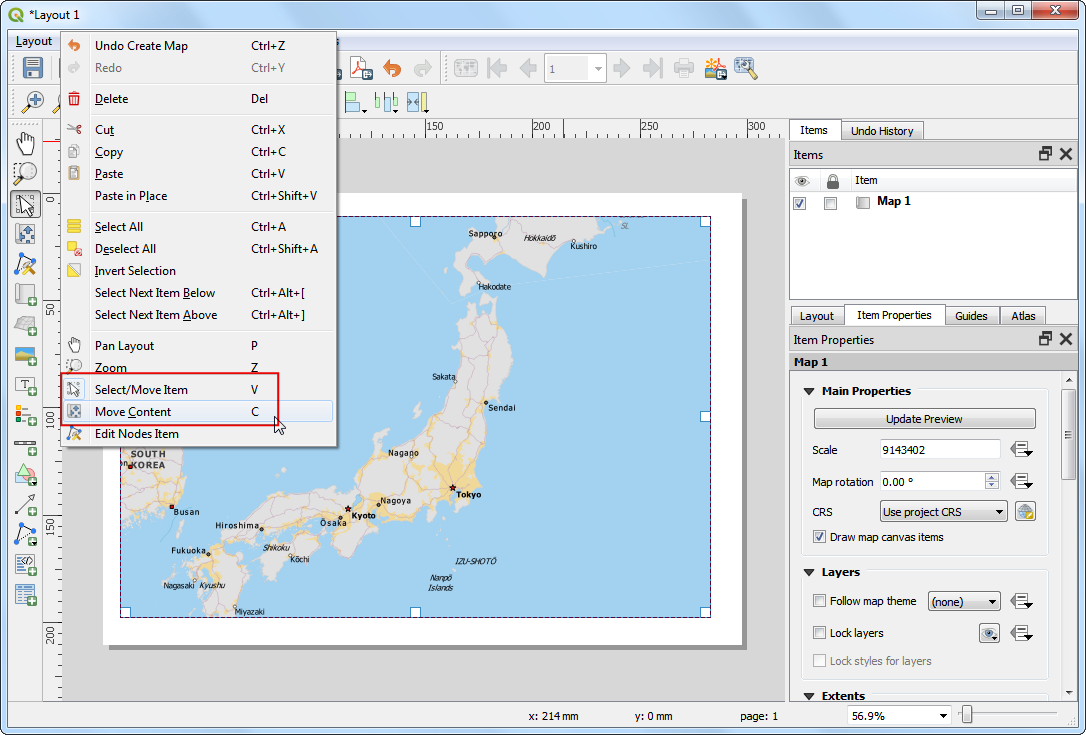1. Load the CSV file. As the CSV file doesn’t contain any spatial data, we load it as a table using the `delimitedtext` provider.
```csv_uri = 'file:///C:/Users/Ujaval/Downloads/ca_tracts_pop.csv?delimiter=,'
csv = QgsVectorLayer(csv_uri, 'ca_tracts_pop', 'delimitedtext')
```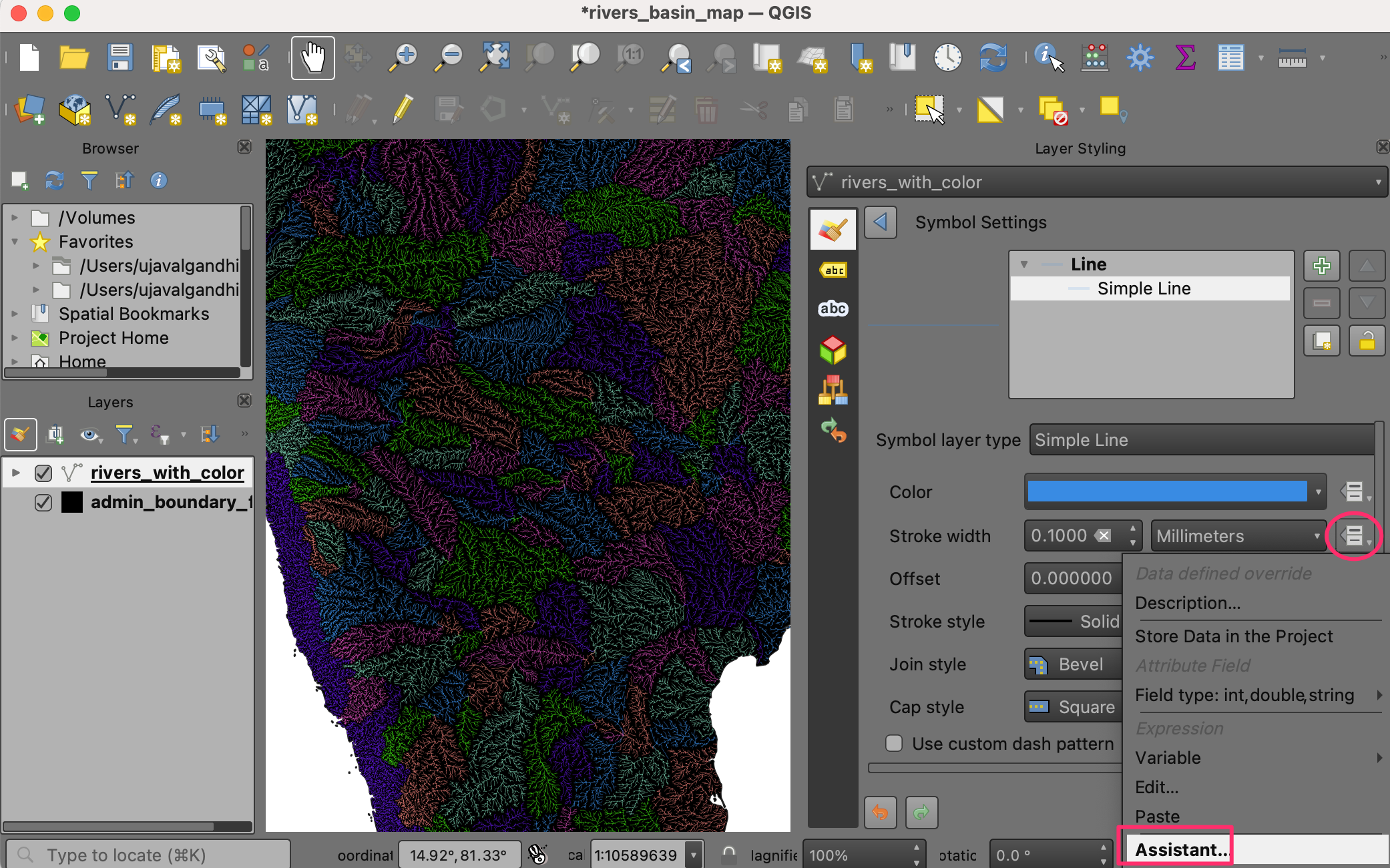1. Create the table join. Table joins in QGIS are performed using `QgsVectorJoinInfo` object. We need to specify the `GEO.id2` field from the CSV layer as the Join Field and the `GEOID` field from the shapefile layer as the Target Field. Once you run the following code, the shapefile layer will have additional attributes joined from the csv layer.

Bemerkung

A common pitfall when using QgsVectorJoinInfo is that both the layers must be loaded in the QgsMapLayerRegistry - otherwise the join would not work.

```shpField='GEOID'
csvField='GEO.id2'
joinObject = QgsVectorJoinInfo()
joinObject.joinLayerId = csv.id()
joinObject.joinFieldName = csvField
joinObject.targetFieldName = shpField
joinObject.memoryCache = True
```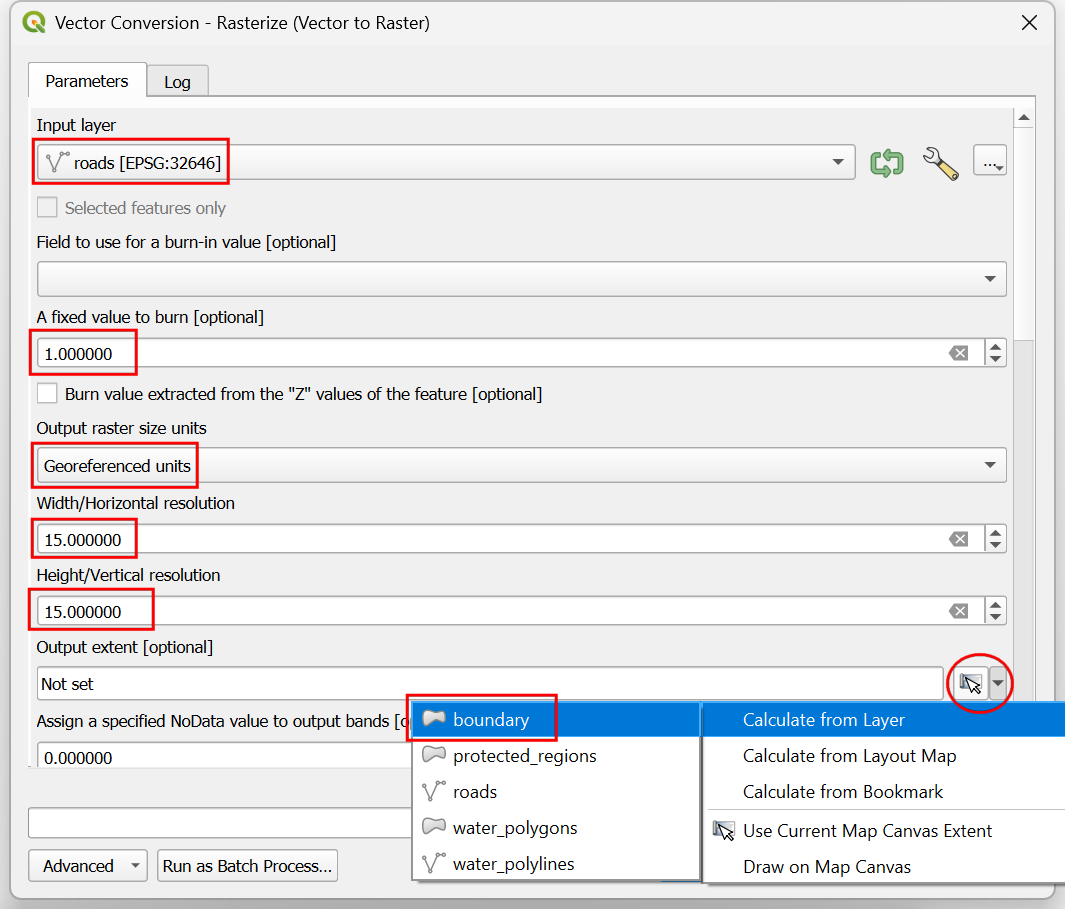1. An easier - and preferred way of accomplishing the same thing is via the Processing Framework. You can call the algorithm `qgis:joinattributestable` and create a joined layer.

Bemerkung

We are using the `processing.runandload()` method to execute the algorithm instead of the more common `processing.runalg()`. Since we want to load the resulting joined layer in QGIS, `processing.runandload()` is a better choice.

```import processing
shpField='GEOID'
csvField='GEO.id2'
result = processing.runandload('qgis:joinattributestable', shp, csv, shpField, csvField, None)
```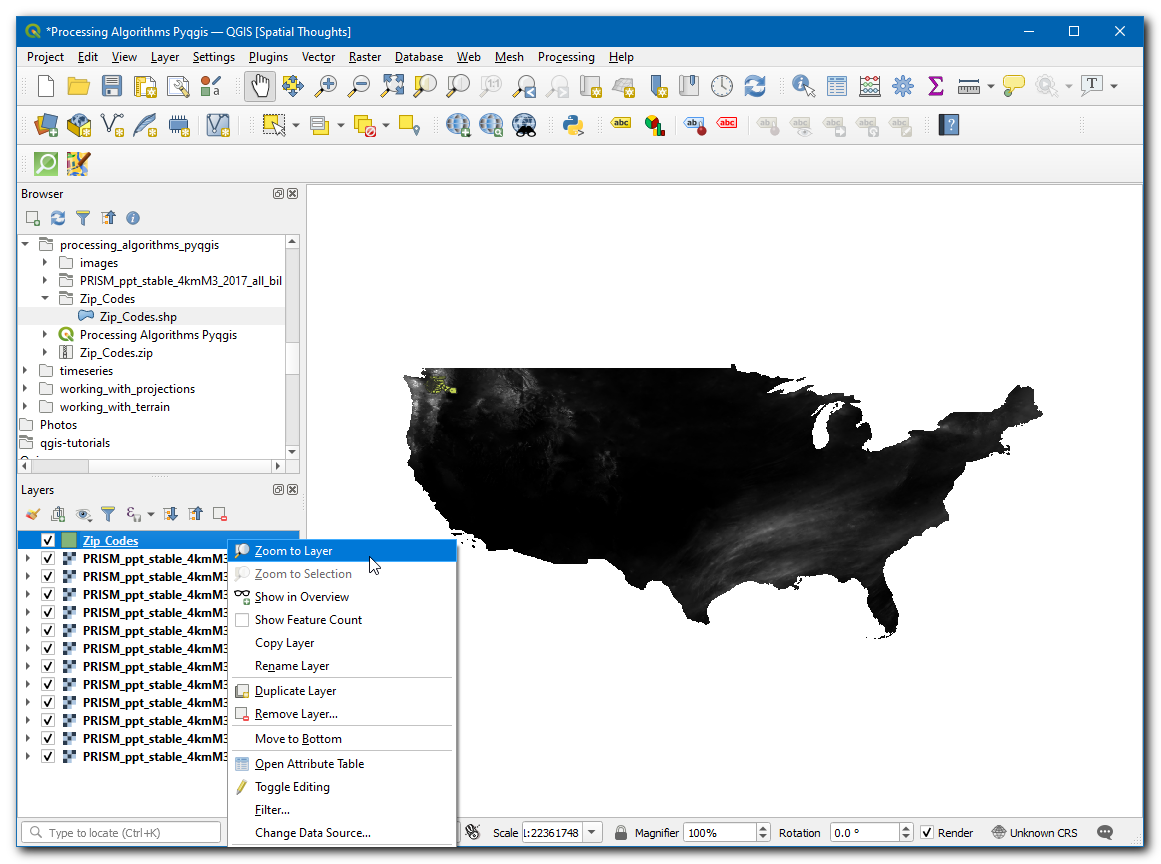1. We will stick with the original join using `QgsVectorJoinInfo` for the remainder of the tutorial. Now it is time to apply a graduated style to the joined layer. The population field name in the joined layer is `ca_tracts_pop_D001`. We will apply a graduated renderer using the `QgsGraduatedSymbolRendererV2` class in the `Quantile` mode. Refer to Performing Table Joins for the colors and ranges that we need to use.
```from PyQt4 import QtGui

myColumn = 'ca_tracts_pop_D001 '
myRangeList = []
myOpacity = 1

ranges = []

myMin1 = 0.0
myMax1 = 3157.2
myLabel1 = 'Group 1'
myColor1 = QtGui.QColor('#f7fbff')
ranges.append((myMin1, myMax1, myLabel1, myColor1))

myMin2 = 3157.2
myMax2 = 4019.0
myLabel2 = 'Group 2'
myColor2 = QtGui.QColor('#c7dcef')
ranges.append((myMin2, myMax2, myLabel2, myColor2))

myMin3 = 4019.0
myMax3 = 4865.8
myLabel3 = 'Group 3'
myColor3 = QtGui.QColor('#72b2d7')
ranges.append((myMin3, myMax3, myLabel3, myColor3))

myMin4 = 4865.8
myMax4 = 5996.4
myLabel4 = 'Group 4'
myColor4 = QtGui.QColor('#2878b8')
ranges.append((myMin4, myMax4, myLabel4, myColor4))

myMin5 = 5996.4
myMax5 = 37452.0
myLabel5 = 'Group 5'
myColor5 = QtGui.QColor('#08306b')
ranges.append((myMin5, myMax5, myLabel5, myColor5))

for myMin, myMax, myLabel, myColor in ranges:
mySymbol = QgsSymbolV2.defaultSymbol(shp.geometryType())
mySymbol.setColor(myColor)
mySymbol.setAlpha(myOpacity)
myRange = QgsRendererRangeV2(myMin, myMax, mySymbol, myLabel)
myRangeList.append(myRange)

myRenderer = QgsGraduatedSymbolRendererV2('', myRangeList)
myRenderer.setClassAttribute(myColumn)

shp.setRendererV2(myRenderer)
```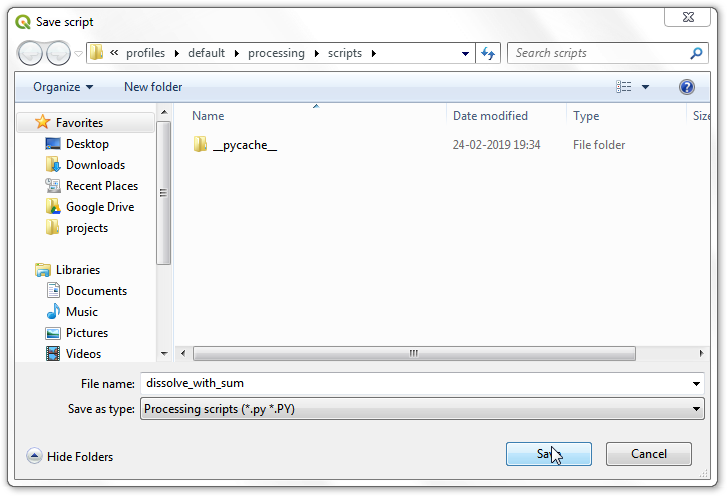1. Typing the code in the Python Console is useful for small tasks, but it is far easier to use the built-in Editor. You can copy the entire script in the Editor and click Run. As the script finishes, you would have created a table join and styled the resulting layer without any manual steps.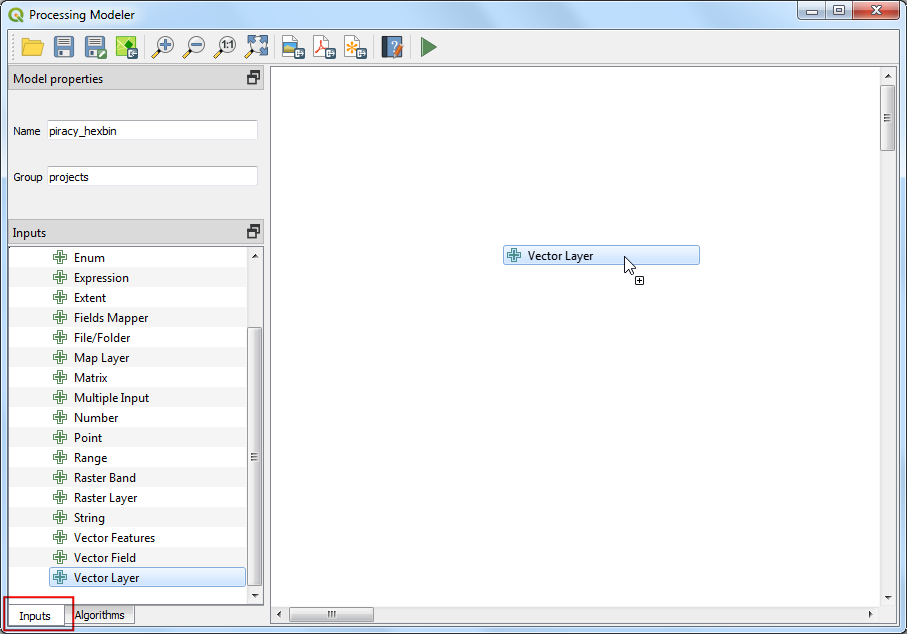Below is the full `join_attributes.py` file as a reference.

```from PyQt4 import QtGui
shp =  QgsVectorLayer(zip_uri, 'tl_2013_06_tract', 'ogr')

csv = QgsVectorLayer(csv_uri, "ca_tracts_pop", "delimitedtext")

shpField='GEOID'
csvField='GEO.id2'
joinObject = QgsVectorJoinInfo()
joinObject.joinLayerId = csv.id()
joinObject.joinFieldName = csvField
joinObject.targetFieldName = shpField
joinObject.memoryCache = True

myColumn = 'ca_tracts_pop_D001 '
myRangeList = []
myOpacity = 1

ranges = []

myMin1 = 0.0
myMax1 = 3157.2
myLabel1 = 'Group 1'
myColor1 = QtGui.QColor('#f7fbff')
ranges.append((myMin1, myMax1, myLabel1, myColor1))

myMin2 = 3157.2
myMax2 = 4019.0
myLabel2 = 'Group 2'
myColor2 = QtGui.QColor('#c7dcef')
ranges.append((myMin2, myMax2, myLabel2, myColor2))

myMin3 = 4019.0
myMax3 = 4865.8
myLabel3 = 'Group 3'
myColor3 = QtGui.QColor('#72b2d7')
ranges.append((myMin3, myMax3, myLabel3, myColor3))

myMin4 = 4865.8
myMax4 = 5996.4
myLabel4 = 'Group 4'
myColor4 = QtGui.QColor('#2878b8')
ranges.append((myMin4, myMax4, myLabel4, myColor4))

myMin5 = 5996.4
myMax5 = 37452.0
myLabel5 = 'Group 5'
myColor5 = QtGui.QColor('#08306b')
ranges.append((myMin5, myMax5, myLabel5, myColor5))

for myMin, myMax, myLabel, myColor in ranges:
mySymbol = QgsSymbolV2.defaultSymbol(shp.geometryType())
mySymbol.setColor(myColor)
mySymbol.setAlpha(myOpacity)
myRange = QgsRendererRangeV2(myMin, myMax, mySymbol, myLabel)
myRangeList.append(myRange)

myRenderer = QgsGraduatedSymbolRendererV2('', myRangeList)